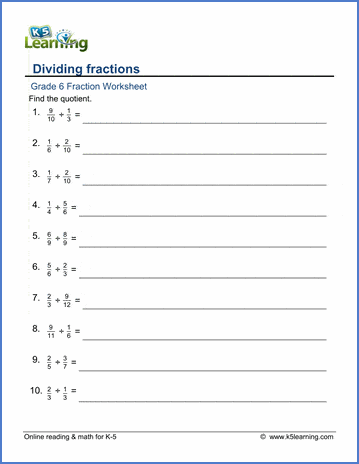# Divide Fractions in Three Simple Steps

In math terms, dividing two fractions is the same as multiplying the first fraction by the reciprocal of the second fraction. You then multiply the two numerators and multiply the two denominators.

Let us show you what that looks like. The easiest way to do that is with an example: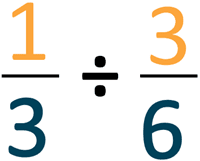## Step 1: Turn the second fraction upside down.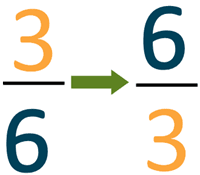This is called the reciprocal.

## Step 2: Multiply the two numbers

Multiple the top numbers (the numerators) and multiply the bottom numbers (the denominators).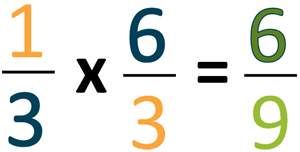## Step 3: simplify the fraction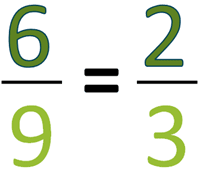In our grade 5 and 6 math sections, we have worksheets to practice dividing fractions by fractions.

## Grade 5 dividing fractions worksheets

These worksheets cover proper fractions for dividing fractions by fractions.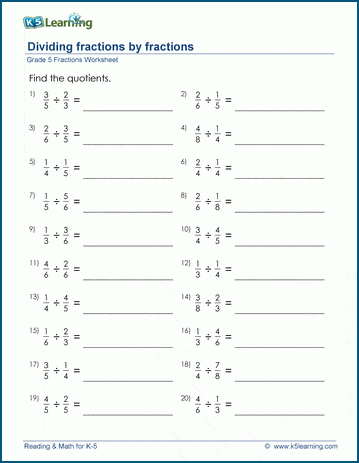## Grade 6 fractions by fractions division worksheets

In these worksheets, students practice dividing proper and improper fractions by other fractions.